Next: 4. Density-Matrix Formulation Up: 3. Quantum Mechanics of Previous: 3.2 Periodic systems   Contents

Subsections

# 3.3 The pseudopotential approximation

In this section we outline a further approximation which is based upon the observation that the core electrons are relatively unaffected by the chemical environment of an atom. Thus we assume that their (large) contribution to the total binding energy does not change when isolated atoms are brought together to form a molecule or crystal. The actual energy differences of interest are the changes in valence electron energies, and so if the binding energy of the core electrons can be subtracted out, the valence electron energy change will be a much larger fraction of the total binding energy, and hence much easier to calculate accurately. We also note that the strong nuclear Coulomb potential and highly localised core electron wave-functions are difficult to represent computationally.

Since the atomic wave-functions are eigenstates of the atomic Hamiltonian, they must all be mutually orthogonal. Since the core states are localised in the vicinity of the nucleus, the valence states must oscillate rapidly in this core region in order to maintain this orthogonality with the core electrons. This rapid oscillation results in a large kinetic energy for the valence electrons in the core region, which roughly cancels the large potential energy due to the strong Coulomb potential. Thus the valence electrons are much more weakly bound than the core electrons.

It is therefore convenient to attempt to replace the strong Coulomb potential and core electrons by an effective pseudopotential which is much weaker, and replace the valence electron wave-functions, which oscillate rapidly in the core region, by pseudo-wave-functions, which vary smoothly in the core region [56,57]. We outline two justifications for this approximation below; for further details see  and also [59,60] for recent reviews.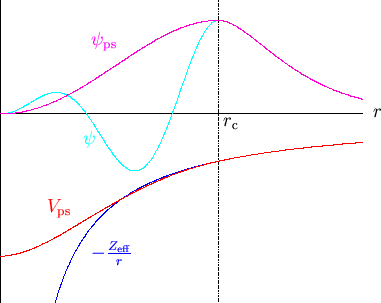## 3.3.1 Operator approach

Following the orthogonalised plane-waves approach , we consider an atom with Hamiltonian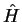, core states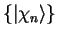and core energy eigenvalues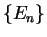and focus on one valence state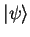with energy eigenvalue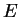. From these states we attempt to construct a smoother pseudo-state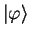defined by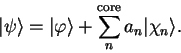(3.55)

The valence state must be orthogonal to all of the core states (which are of course mutually orthogonal) so that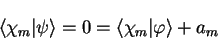(3.56)

which fixes the expansion coefficients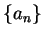. Thus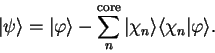(3.57)

Substituting this expression in the Schrödinger equation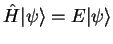gives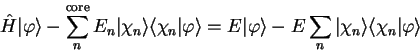(3.58)

which can be rearranged in the form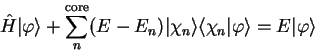(3.59)

so that the smooth pseudo-state obeys a Schrödinger equation with an extra energy-dependent non-local potential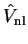;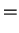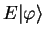(3.60)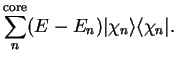(3.61)

The energy of the smooth state described by the pseudo-wave-function is the same as that of the original valence state. The additional potential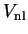, whose effect is localised in the core, is repulsive and will cancel part of the strong Coulomb potential so that the resulting sum is a weaker pseudopotential. Of course, once the atom interacts with others, the energies of the eigenstates will change, but if the core states are reasonably far from the valence states in energy (i.e.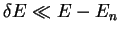) then fixinginto be the atomic valence eigenvalue is a reasonable approximation. In fact we would like to make the behaviour of the pseudopotential follow that of the real potential to first order in, and this can be achieved by constructing a norm-conserving pseudopotential (see section 3.3.3).

## 3.3.2 Scattering approach

For a fuller discussion of the theory of scattering see . Consider a plane-wave with wave-vector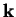scattering from some spherically-symmetric potential localised within a radius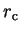and centred at the origin. The incoming plane-wave can be decomposed into spherical-waves by the identity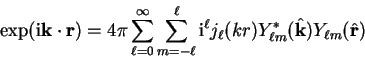(3.62)

where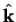denotes a unit vector in the direction of. These spherical- or partial-waves are then elastically scattered by the potential which introduces a phase-shift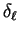, which is related to the logarithmic derivative of the exact radial solution for givenand energy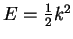within the core, evaluated on the surface of the core region: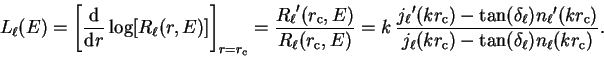(3.63)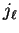and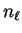denote the spherical Bessel and von Neumann functions respectively, and the radial wave-function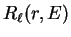is related to the solution of the Schrödinger equation with angular momentum state determined by the good quantum numbersand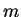, and energy, within the core region,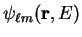by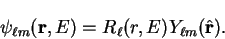(3.64)

The phase-shifted spherical-waves can then be recombined to form the total scattered wave. We can define a reduced phase-shift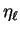by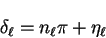(3.65)

which has the same effect (the scattering amplitude depends on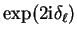so that factors of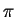inhave no effect) and fixby requiringto lie in the interval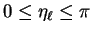. The integercounts the number of radial nodes in, two in the case of figure 3.2, and is thus equal to the number of core states with angular momentum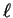.

The pseudopotential is then defined as the potential whose complete phase-shifts are the reduced shiftsso that the radial pseudo-wave-function has no nodes and thus the potential has no core states. The scattering effect of this potential is the same as the original potential. We note again the energy-dependence of the phase-shifts so that for a good approximation it will be necessary to match these phase-shifts to first order in the energy so that it is accurate over a reasonable range of energies, a property which results in good transferability of the pseudopotential i.e. it is accurate in a variety of different chemical environments. The non-local nature is also exhibited since different angular momentum states are scattered differently.

## 3.3.3 Norm conservation

The conditions of a good pseudopotential are that it reproduces the logarithmic derivative of the wave-function (and thus the phase-shifts) correctly for the isolated atom, and also that the variation of this quantity with respect to energy is the same to first order for pseudopotential and full potential3.2. Having replaced the full potential by a pseudopotential, we can once again solve the Schrödinger equation in the core region to obtain the pseudo-wave-function, with radial part.

Pseudopotential generation has itself been the subject of a great deal of study in the past (see [65,66,67,68,69,70,71,72,73]) and in this work we have chosen to use those pseudopotentials generated by the method of Troullier and Martins . With one notable exception , all of the recent methods have used norm-conservation to guarantee that the phase-shifts are correct to first order in the energy (correction to higher orders is also possible ).

Consider the following second-order ordinary differential equations which are eigenvalue equations for the same differential operator but with different eigenvalues: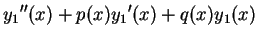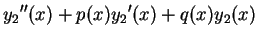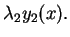(3.66)

In the context of homogeneous differential equations, the quantity known as the Wronskian is defined by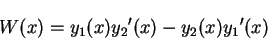(3.67)

and can be calculated according to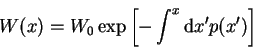(3.68)

in which the constant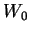is arbitrary and of no consequence.

Following a similar analysis which leads to equation 3.68 for the quantity defined in equation 3.67 but for the functions which solve equations 3.66 we obtain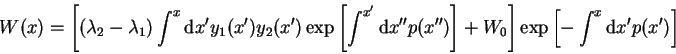(3.69)

and note that the Wronskian can also be rewritten in terms of logarithmic derivatives: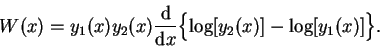(3.70)

Using equations 3.69 and 3.70 in the case of the Schrödinger equation for the radial wave-function, by making the replacements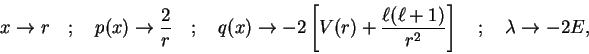and using limits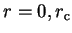we obtain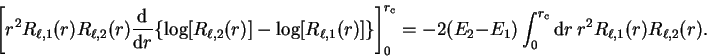(3.71)

Rearranging, multiplying by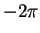and noting that the lower limit on the left-hand side contributes nothing because of the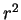factor: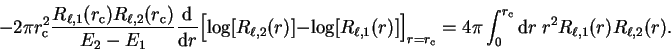(3.72)

Finally, taking the limit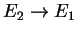so that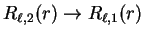and interpreting the left-hand side as a derivative with respect to energy we obtain the desired result: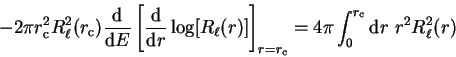(3.73)

i.e. the first energy-derivative of the logarithmic derivative evaluated at the core radius (and hence the phase-shift) is related directly to the norm of the radial wave-function within the core region. Thus if the pseudo-wave-function is norm-conserving such that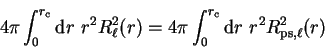(3.74)

then the phase-shifts of the pseudopotential will be the same as those of the real potential to first order in energy, and this can be achieved by making the pseudo-wave-function identical to the original all-electron wave-function outside the core region.

## 3.3.4 Kleinman-Bylander representation

We have seen that it is necessary to use a non-local pseudopotential to accurately represent the combined effect of nucleus and core electrons, since different angular momentum states (partial waves) are scattered differently. In general we can express the non-local pseudopotential in semi-local form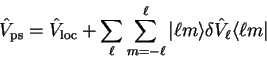(3.75)

in which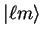denotes the spherical harmonic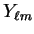. The choice of local potential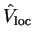is arbitrary, but in general the sum overis truncated at a small value (e.g.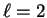) so that the local part is required to represent the potential which acts on higher angular momentum components.

This semi-local form suffers from the disadvantage that it is computationally very expensive to use, since the number of matrix elements which need to be calculated scales as the square of the number of basis states, and this is generally too costly. In section 5.6.1 we will describe how this problem can be overcome analytically with a certain set of localised basis functions, but the most common solution, and one which we have also implemented for consistency, is to use the Kleinman-Bylander separable form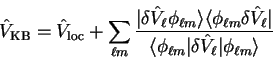(3.76)

where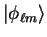is an eigenstate of the atomic pseudo-Hamiltonian. This operator acts on this reference state in an identical manner to the original semi-local operator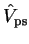so that it is conceptually well-justified, but now the number of projections which need to be performed scales only linearly with the number of basis states. This separable form can in fact be viewed as the first term of a complete series .Next: 4. Density-Matrix Formulation Up: 3. Quantum Mechanics of Previous: 3.2 Periodic systems   Contents
Peter Haynes